# Welcome to the DFA Minimization MCQs Page

Dive deep into the fascinating world of DFA Minimization with our comprehensive set of Multiple-Choice Questions (MCQs). This page is dedicated to exploring the fundamental concepts and intricacies of DFA Minimization, a crucial aspect of Formal Languages and Automata Theory. In this section, you will encounter a diverse range of MCQs that cover various aspects of DFA Minimization, from the basic principles to advanced topics. Each question is thoughtfully crafted to challenge your knowledge and deepen your understanding of this critical subcategory within Formal Languages and Automata Theory.

Check out the MCQs below to embark on an enriching journey through DFA Minimization. Test your knowledge, expand your horizons, and solidify your grasp on this vital area of Formal Languages and Automata Theory.

Note: Each MCQ comes with multiple answer choices. Select the most appropriate option and test your understanding of DFA Minimization. You can click on an option to test your knowledge before viewing the solution for a MCQ. Happy learning!

### DFA Minimization MCQs | Page 1 of 2

Q1.
Given a NFA with N states, the maximum number of states in an equivalent minimized DFA is at least
Q2.
Myhill-Nerode Theorem is used for __________
Q3.
A deterministic finite automation (DFA)D with alphabet ∑= {a,b} is given below. Which of the following is a valid minimal DFA which accepts the same language as D?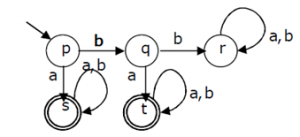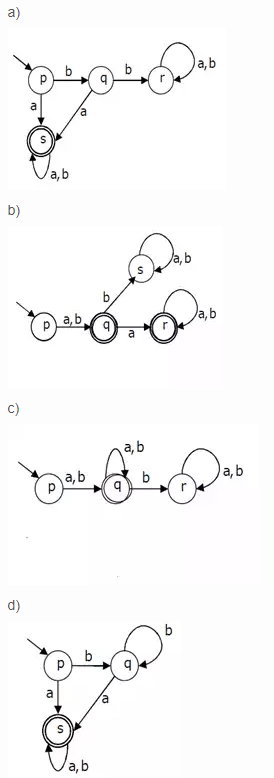a.

a

b.

b

c.

c

d.

d

Q4.
Consider the languages L1 = and L2 = {a}. Which one of the following represents L1 L2* U L1* .
Q5.
Choose the correct statement for the following: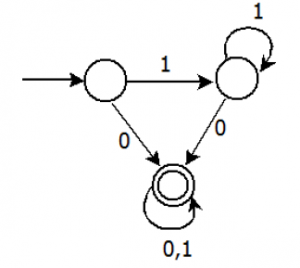Q6.
Given the language L = {ab, aa, baa}, which of the following strings are in L* ?

1) abaabaaabaa
2) aaaabaaaa
3) baaaaabaaaab
4) baaaaabaa
Q7.
What is the complement of the language accepted by the NFA shown below?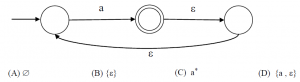Q8.
Which one of the following is FALSE?
Answer: (b).Every subset of a recursively enumerable set is recursive
Q9.
The language accepted by this DFA isQ10.
The minimum state automaton equivalent to the below FSA has the following number of states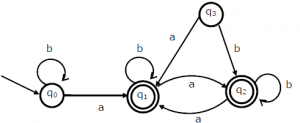a.

1

b.

2

c.

3

d.

4